# 5.5 Solve mixture applications with systems of equations  (Page 4/7)

 Page 4 / 7

Translate to a system of equations and solve:

Anatole needs to make 250 milliliters of a 25% solution of hydrochloric acid for a lab experiment. The lab only has a 10% solution and a 40% solution in the storeroom. How much of the 10% and how much of the 40% solutions should he mix to make the 25% solution?

Anatole should mix 125 ml of the 10% solution and 125 ml of the 40% solution.

## Solve interest applications

The formula to model interest applications is I = Prt . Interest, I , is the product of the principal, P , the rate, r , and the time, t . In our work here, we will calculate the interest earned in one year, so t will be 1.

We modify the column titles in the mixture table to show the formula for interest, as you’ll see in [link] .

Translate to a system of equations and solve:

Adnan has $40,000 to invest and hopes to earn 7.1% interest per year. He will put some of the money into a stock fund that earns 8% per year and the rest into bonds that earns 3% per year. How much money should he put into each fund? ## Solution  Step 1. Read the problem. A chart will help us organize the information. Step 2. Identify what we are looking for. We are looking for the amount to invest in each fund. Step 3. Name what we are looking for. Let $s=$ the amount invested in stocks. $\phantom{\rule{1.5em}{0ex}}b=$ the amount invested in bonds. Write the interest rate as a decimal for each fund. Multiply: Principal · Rate · Time to get the Interest.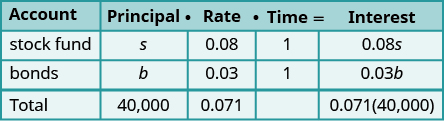Step 4. Translate into a system of equations. We get our system of equations from the Principal column and the Interest column.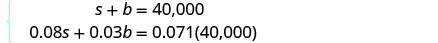Step 5. Solve the system of equations Solve by elimination. Multiply the top equation by −0.03.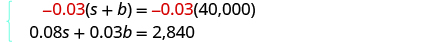Simplify and add to solve for s .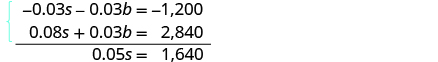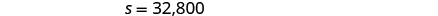To find b , substitute s = 32,800 into the first equation.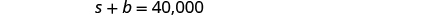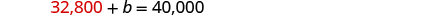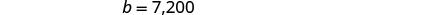Step 6. Check the answer in the problem. We leave the check to you. Step 7. Answer the question. Adnan should invest$32,000 in stock and $7,200 in bonds. Did you notice that the Principal column represents the total amount of money invested while the Interest column represents only the interest earned? Likewise, the first equation in our system, s + b = 40,000, represents the total amount of money invested and the second equation, 0.08 s + 0.03 b = 0.071(40,000), represents the interest earned. Translate to a system of equations and solve: Leon had$50,000 to invest and hopes to earn 6.2 % interest per year. He will put some of the money into a stock fund that earns 7% per year and the rest in to a savings account that earns 2% per year. How much money should he put into each fund?

Leon should put $42,000 in the stock fund and$8000 in the savings account.

Translate to a system of equations and solve:

Julius invested $7,000 into two stock investments. One stock paid 11% interest and the other stock paid 13% interest. He earned 12.5% interest on the total investment. How much money did he put in each stock? Julius invested$1,750 at 11% and $5,250 at 13%. Translate to a system of equations and solve: Rosie owes$21,540 on her two student loans. The interest rate on her bank loan is 10.5% and the interest rate on the federal loan is 5.9%. The total amount of interest she paid last year was $1,669.68. What was the principal for each loan? ## Solution  Step 1. Read the problem. A chart will help us organize the information. Step 2. Identify what we are looking for. We are looking for the principal of each loan. Step 3. Name what we are looking for. Let $b=$ the principal for the bank loan. $\phantom{\rule{1.5em}{0ex}}f=$ the principal on the federal loan The total loans are$21,540. Record the interest rates as decimals in the chart.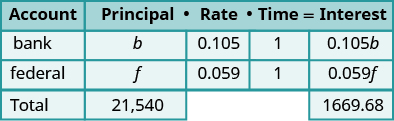Multiply using the formula l = Pr t to get the Interest. Step 4. Translate into a system of equations. The system of equations comes from the Principal column and the Interest column.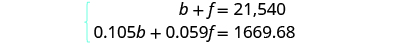Step 5. Solve the system of equations We will use substitution to solve. Solve the first equation for b .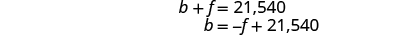Substitute b = − f + 21,540 into the second equation.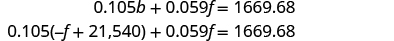Simplify and solve for f .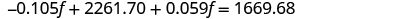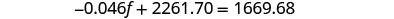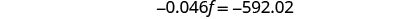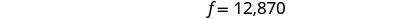To find b , substitute f = 12,870 into the first equation.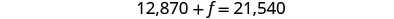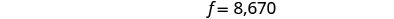Step 6. Check the answer in the problem. We leave the check to you. Step 7. Answer the question. The principal of the bank loan is $12,870 and the principal for the federal loan is$8,670.

the proudact of 3x^3-5×^2+3 and 2x^2+5x-4 in z7[x]/ is
?
Choli
a rock is thrown directly upward with an initial velocity of 96feet per second from a cliff 190 feet above a beach. The hight of tha rock above the beach after t second is given by the equation h=_16t^2+96t+190
Usman
Stella bought a dinette set on sale for $725. The original price was$1,299. To the nearest tenth of a percent, what was the rate of discount?
44.19%
Scott
40.22%
Terence
44.2%
Orlando
p mulripied-5 and add 30 to it
Tausif
Tausif
Can you explain further
p mulripied-5 and add to 30
Tausif
How do you find divisible numbers without a calculator?
TAKE OFF THE LAST DIGIT AND MULTIPLY IT 9. SUBTRACT IT THE DIGITS YOU HAVE LEFT. IF THE ANSWER DIVIDES BY 13(OR IS ZERO), THEN YOUR ORIGINAL NUMBER WILL ALSO DIVIDE BY 13!IS DIVISIBLE BY 13
BAINAMA
When she graduates college, Linda will owe $43,000 in student loans. The interest rate on the federal loans is 4.5% and the rate on the private bank loans is 2%. The total interest she owes for one year was$1,585. What is the amount of each loan?
Sean took the bus from Seattle to Boise, a distance of 506 miles. If the trip took 7 2/3 hours, what was the speed of the bus?
66miles/hour
snigdha
How did you work it out?
Esther
s=mi/hr 2/3~0.67 s=506mi/7.67hr = ~66 mi/hr
Orlando
hello, I have algebra phobia. Subtracting negative numbers always seem to get me confused.
what do you need help in?
Felix
Heather
look at the numbers if they have different signs, it's like subtracting....but you keep the sign of the largest number...
Felix
for example.... -19 + 7.... different signs...subtract.... 12 keep the sign of the "largest" number 19 is bigger than 7.... 19 has the negative sign... Therefore, -12 is your answer...
Felix
—12
Thanks Felix.l also get confused with signs.
Esther
Thank you for this
Shatey
ty
Graham
think about it like you lost $19 (-19), then found$7(+7). Totally you lost just $12 (-12) Annushka I used to struggle a lot with negative numbers and math in general what I typically do is look at it in terms of money I have -$5 in my account I then take out 5 more dollars how much do I have in my account well-\$10 ... I also for a long time would draw it out on a number line to visualize it
Meg
practicing with smaller numbers to understand then working with larger numbers helps too and the song/rhyme same sign add and keep opposite signs subtract keep the sign of the bigger # then you'll be exact
Meg
Bruce drives his car for his job. The equation R=0.575m+42 models the relation between the amount in dollars, R, that he is reimbursed and the number of miles, m, he drives in one day. Find the amount Bruce is reimbursed on a day when he drives 220 miles
168.50=R
Heather
john is 5years older than wanjiru.the sum of their years is27years.what is the age of each
46
mustee
j 17 w 11
Joseph
john is 16. wanjiru is 11.
Felix
27-5=22 22÷2=11 11+5=16
Joyce
I don't see where the answers are.
Ed
Cindy and Richard leave their dorm in Charleston at the same time. Cindy rides her bicycle north at a speed of 18 miles per hour. Richard rides his bicycle south at a speed of 14 miles per hour. How long will it take them to be 96 miles apart?
3
Christopher
18t+14t=96 32t=96 32/96 3
Christopher
show that a^n-b^2n is divisible by a-b
What does 3 times your weight right now
Use algebra to combine 39×5 and the half sum of travel of 59+30
Cherokee
What is the segment of 13? Explain
Cherokee
my weight is 49. So 3 times is 147
Cherokee
kg to lbs you goin to convert 2.2 or one if the same unit your going to time your body weight by 3. example if my body weight is 210lb. what would be my weight if I was 3 times as much in kg. that's you do 210 x3 = 630lb. then 630 x 2.2= .... hope this helps
tyler
How to convert grams to pounds?
paul
What is the lcm of 340
Yes
CherokeeBy Vanessa SoledadBy Madison ChristianBy OpenStaxBy OpenStaxBy Brooke DelaneyBy OpenStaxBy Jazzycazz JacksonBy Janet ForresterByBy Anonymous User